# Norton/Thevenin Equivalent circuits with a dependent voltage source

• Engineering
• RED119
In summary, the conversation discusses the process of finding the Thevenin and Norton equivalents of a circuit. It includes a problem where the answer obtained through source transformations does not match the answer obtained by shorting the leads, leading to a discussion on the various methods for finding Rth and the potential for algebraic errors. The conversation also addresses the issue of dependent sources and how to handle them in finding the Thevenin and Norton equivalents. Ultimately, it is suggested to verify the algebraic solution and to approach the professor for clarification before making any conclusions.

#### RED119

Homework Statement
Find the thevenin and norton equivalents of the circuit.
Relevant Equations
KCL, Nodal Analysis, Thevenin and Norton Equivalents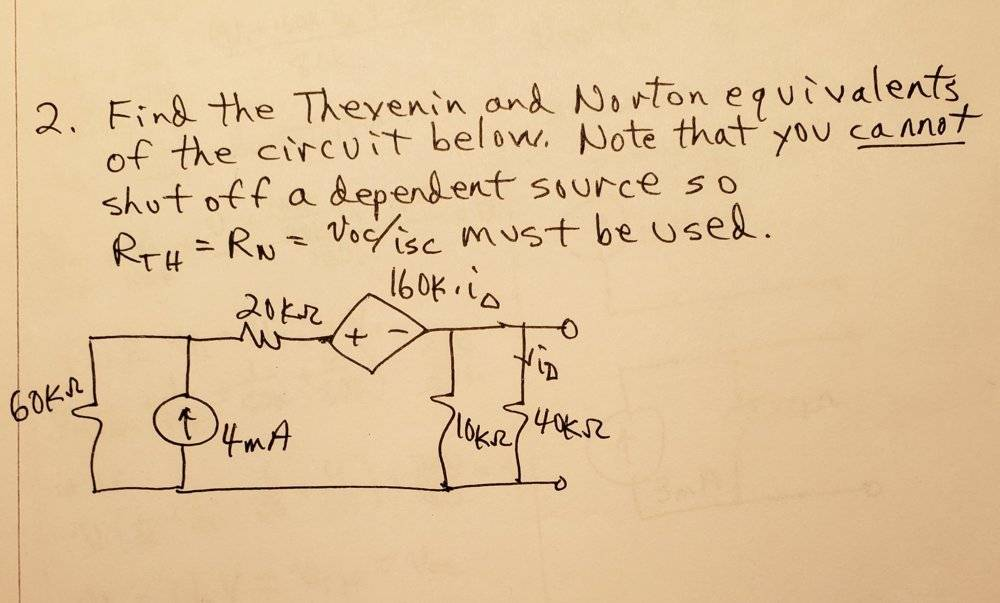so when I did this problem I did nodal analysis to find the voltage across the 40k resistor, and found it to be 16V. From there I did two source transformations, combined the sources and did some equivalent resistance to get the answer as seen below: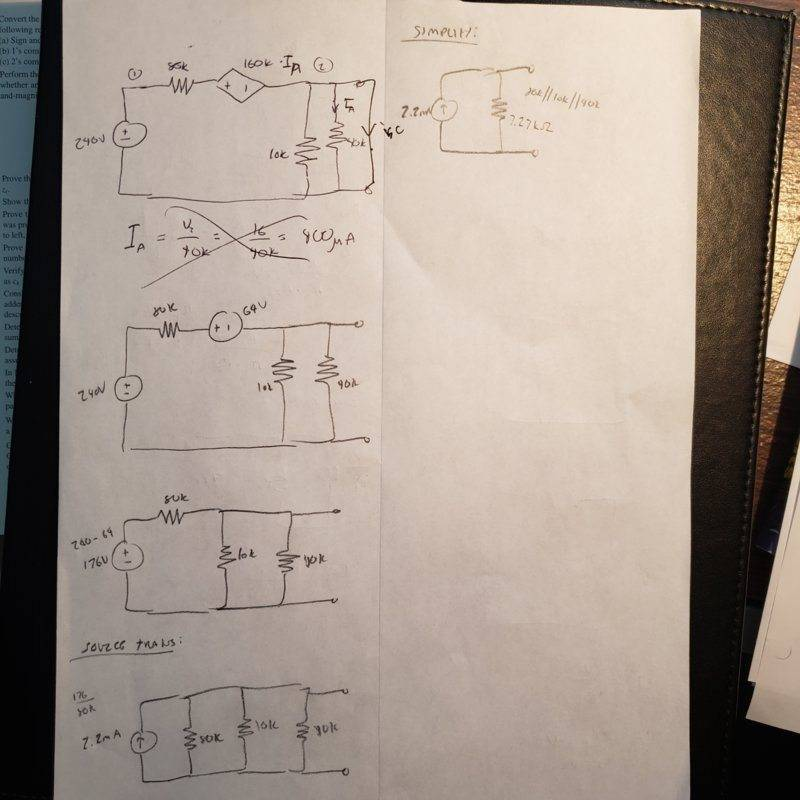However this differed from the answer he gave which was 3mA with a 5.33k resistor as seen below: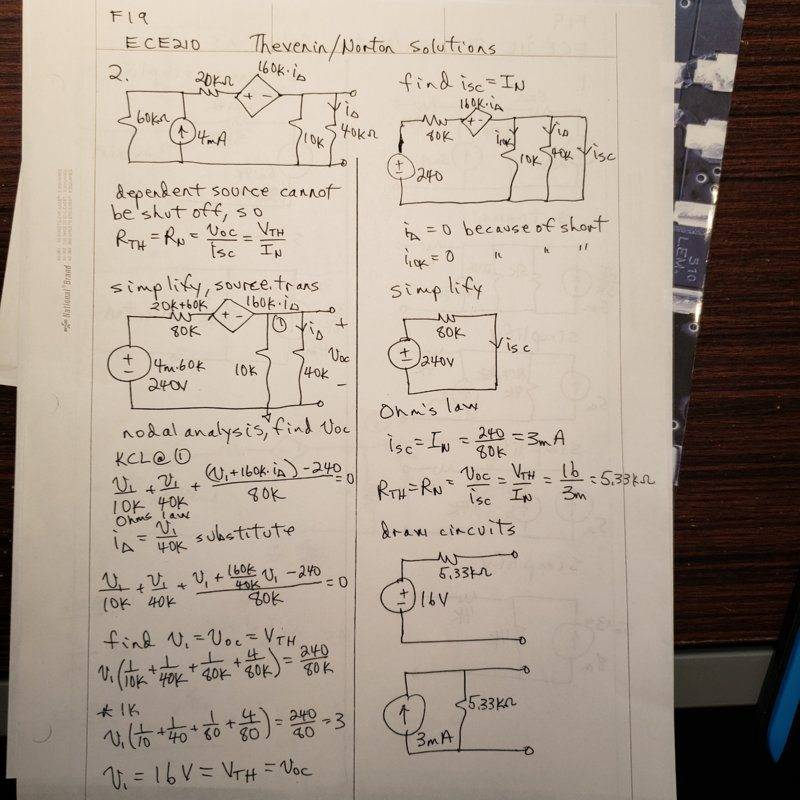So when I went to check and look at it I think the answer may be wrong, both that is. You have a current source that is dependent on IA, however IA is dependent on what the circuit is hooked up to, as you essentially have a current divider. So the current is going to be dependent on the circuit attached, as is the dependent source, so it seems to be like the answer should be a function as opposed to a hard answer.The essence of Thevenin and Norton equivalents is that they are a way to simplify the circuit but be functionally equivalent. So to verify this I did out a "test" of sorts by hooking up the circuit, both in its original form and its Thevenin and Norton equivalents, at an arbitrary resistor (I chose 1k). These three should be functionally equivalent with respect to the 1k resistor, however as seen below that is not what I found: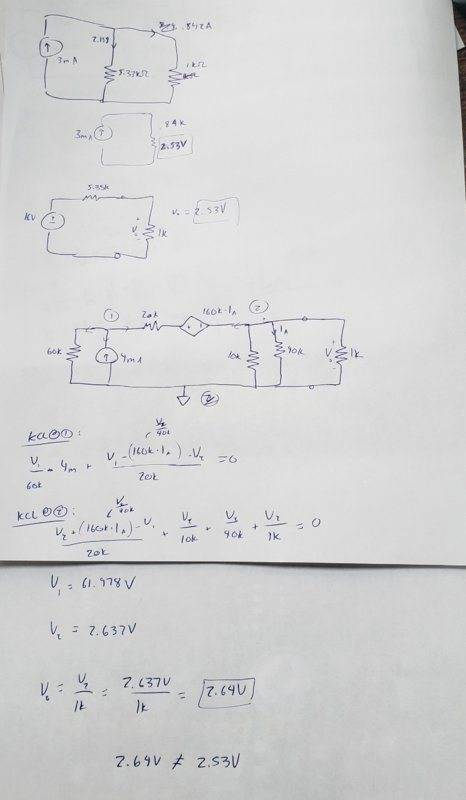I did out nodal analysis for the equivalent circuit and got a different voltage across the 1k resistor than when I used the Norton/Thevenin equivalents. I think, as I mentioned before, this is due to the dependency of the voltage source on the current which can be affected by what is hooked up to the circuit, meaning that the answer should be a function rather than a simple number, as dependent upon the resistance of the circuit hooked up it could affect the internal equivalency of the circuit.

I just wanted to post on here, where all these really big brain physics people reside, before I approach my professor about it and make sure my thinking is right. Cause I may very-well be wrong here, certainly wouldn't be the first time. Thanks for reading and sorry for the bad photos.

RED119 said:
Homework Statement: Find the thevenin and norton equivalents of the circuit.
Homework Equations: KCL, Nodal Analysis, Thevenin and Norton Equivalents

so when I did this problem I did nodal analysis to find the voltage across the 40k resistor, and found it to be 16V
That is correct. This is your Vth.

For Rth, you can see your professor's solution on the right side of the sheet.

Once you have Rth and Vth, you can easily convert it into the Norton equivalent.

cnh1995 said:
That is correct. This is your Vth.

For Rth, you can see your professor's solution on the right side of the sheet.

Once you have Rth and Vth, you can easily convert it into the Norton equivalent.
Right but source transformations/simplification should come out equivalent to the Norton and Thevenin equivalents, which they did not. The Thevenin and Norton equivalents do not match with the actual circuit when it is hooked up to another resistor, which they should. I do not think the standard method of shorting the output leads works here since that is finding the Norton equivalent in a particular instance, given the dependency on IA. If IA was buried within the circuit, at a different node, then shorting the leads and source transformation/simplification would come out equivalent.

Ok I see your problem. You want to verify whether both Thevenin and Norton give you the same result as other methods.

I believe there is an algebraic error in your solution. Try using the source transformation as your professor did. Minimizing the loops always helps, especially when there are current sources and dependent sources in the circuit.

RED119 said:
I do not think the standard method of shorting the output leads works here since that is finding the Norton equivalent in a particular instance, given the dependency on IA.
Actually, this is one of the two methods you can use when dealing with dependent sources. Shorting the output leads is absolutely fine here as you are not "physically" disabling the dependent source. When you short the output leads, the dependent source also gets shorted by means of its transfer function.

The other method for finding Rth is by putting a voltage source of known magnitude (usually 1V) across the output leads and calculating the current supplied by the source. The ratio Vin/Iin gives the Thevenin resistance viewed from the leads.

cnh1995 said:
Actually, this is one of the two methods you can use when dealing with dependent sources. Shorting the output leads is absolutely fine here as you are not "physically" disabling the dependent source. When you short the output leads, the dependent source also gets shorted by means of its transfer function.

The other method for finding Rth is by putting a voltage source of known magnitude (usually 1V) across the output leads and calculating the current supplied by the source. The ratio Vin/Iin gives the Thevenin resistance viewed from the leads.
But doing source transformations/simplification after you algebraically solve for the current and calculate the voltage of the dependent source should aslo work, right?

For example this algebraic example, you need to find the norton and thevenin equivalents of the circuit shown in the top right. So I tried doing it both ways, shorting the leads, which yielded a thevenin/norton resistance of R1 and R2 in parallel, and a norton current of Vs/R1. And if you do it out using a source transformation you get the same thing, as can be seen at the bottom right, a current source of Vs/R1, with a thevenin/norton resistance of R1 || R2. So the algebra works out in doing it both ways.

So why would the answer gotten from doing source transformations be different from shorting the leads? I think its because the circuit in the aforementioned problem is internally altered by the circuit attached to it, whereas if I did the same thing as done in photo 3 of the first post on this example below, then it would be the exact same answer for the original circuit and both equivalents. Not sure if that was clear at all.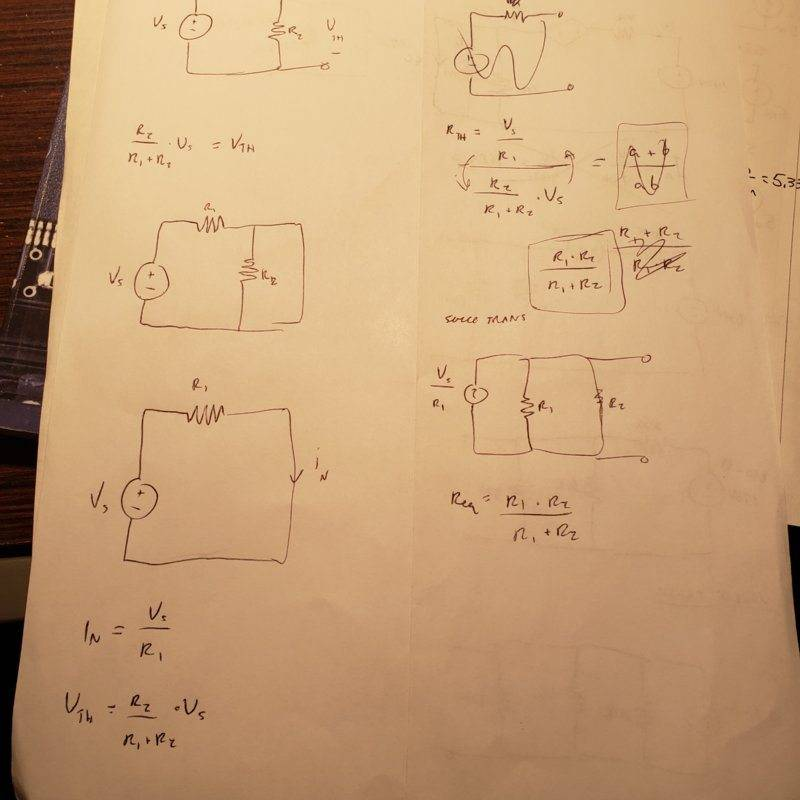RED119 said:
However this differed from the answer he gave which was 3mA with a 5.33k resistor as seen below

For the first part of your demo, I have the following observation to your reasoning.

You assume that the output is constant 16V and then replace the current dependent voltage source by constant 64V, therefore your final simplied circuit also denot the output = 2.2mA * 7.27k = 16V, it is consistent without problem.

However, the whole circuit is already not the Thevenin Equivalent to the original, it is because the output of Thevenin Equivalent circuit is not constant 16V, but depends on the external loading network.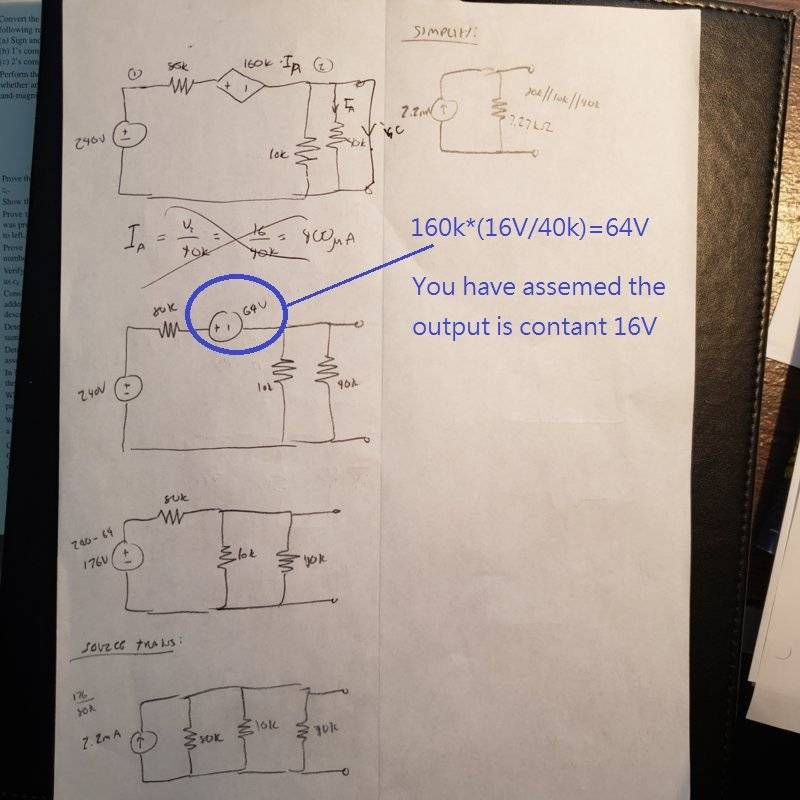Last edited:
•cnh1995
alan123hk said:
You assume that the output is constant 16V and then replace the current dependent voltage source by constant 64V,
Good observation!

I was too lazy to look into the working and point out the exact error.

Definitions of Thevenin's theorem and Norton's theorem : -http://www.angelfire.com/electronic2/jgaress/thevenin.html

By definition, we need to find VTH and RTH to form the equivalent circuit of Thevenin, and find IN and RN to form Norton's equivalent circuit.

The mentioned process of replacing the current-dependent voltage source with a constant 64V based on the open circuit voltage 16V is not suitable for the case. in contrast, the definitions require that all sources be reduced to zero when finding RTH and RN.

Last edited:
alan123hk said:
However, the whole circuit is already not the Thevenin Equivalent to the original, it is because the output of Thevenin Equivalent circuit is not constant 16V, but depends on the external loading network

This is the essence of my question. Because the circuit depends on the "external loading network" is it even possible to create a thevenin equivalent that isn't a function of what you connect to it?

I get that this would be completely possible if the voltage source was dependent upon the internal network, but for a dependency on what you hook up to it, that's where I am unsure. thanks for the responses.